Download Presentation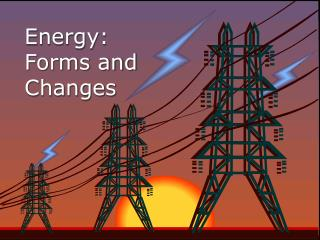Energy: Forms and Changes

# Energy: Forms and Changes - PowerPoint PPT Presentation

Energy: Forms and Changes. Nature of Energy. Energy is all around you! You can hear energy as sound. You can see energy as light. And you can feel it as wind. Nature of Energy. Energy is the ability to do work or bring about change. Nature of Energy.I am the owner, or an agent authorized to act on behalf of the owner, of the copyrighted work described.
Download Presentation## Energy: Forms and Changes

An Image/Link below is provided (as is) to download presentation

Download Policy: Content on the Website is provided to you AS IS for your information and personal use and may not be sold / licensed / shared on other websites without getting consent from its author.While downloading, if for some reason you are not able to download a presentation, the publisher may have deleted the file from their server.

- - - - - - - - - - - - - - - - - - - - - - - - - - E N D - - - - - - - - - - - - - - - - - - - - - - - - - -
Presentation Transcript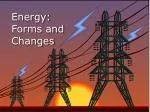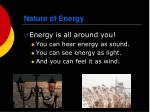Nature of Energy
• Energy is all around you!
• You can hear energy as sound.
• You can see energy as light.
• And you can feel it as wind.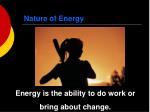Nature of Energy

Energy is the ability to do work or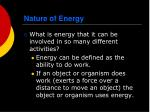Nature of Energy
• What is energy that it can be involved in so many different activities?
• Energy can be defined as the ability to do work.
• If an object or organism does work (exerts a force over a distance to move an object) the object or organism uses energy.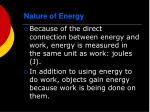Nature of Energy
• Because of the direct connection between energy and work, energy is measured in the same unit as work: joules (J).
• In addition to using energy to do work, objects gain energy because work is being done on them.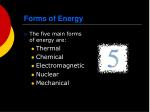Forms of Energy
• The five main forms of energy are:
• Thermal
• Chemical
• Electromagnetic
• Nuclear
• Mechanical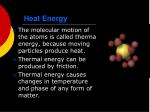Heat Energy
• The molecular motion of the atoms is called thermal energy, because moving particles produce heat.
• Thermal energy can be produced by friction.
• Thermal energy causes changes in temperature and phase of any form of matter.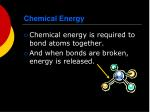Chemical Energy
• Chemical energy is required to bond atoms together.
• And when bonds are broken, energy is released.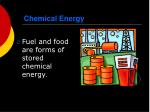Chemical Energy
• Fuel and food are forms of stored chemical energy.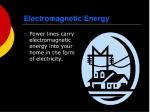Electromagnetic Energy
• Power lines carry electromagnetic energy into your home in the form of electricity.Electromagnetic Energy
• Light is a form of electromagnetic energy.
• Each color of light (Roy G Biv) represents a different amount of electromagnetic energy.
• Electromagnetic Energy is also carried by X-rays, radio waves, and laser light.Nuclear Energy
• The nucleus of an atom is the source of nuclear energy.Nuclear Energy
• When the nucleus splits—fission— nuclear energy is released in the form of thermal energy and EM energy.
• Nuclear energy is also released when nuclei collide at high speeds and undergo fusionNuclear Energy

The sun’s energy is produced from nuclear fusion in which hydrogen nuclei fuse to form helium nuclei.Nuclear Energy
• Nuclear energy is the most concentrated form of energy.Mechanical Energy
• When work is done to an object, it acquires energy. The energy it acquires is known as mechanical energy.Mechanical Energy
• When you kick a football, you give mechanical energy to the football to make it move.Mechanical Energy

When you throw a bowling ball, you give it energy. When that bowling ball hits the pins, some of the energy is transferred to the pins.Energy Conversion
• Energy can be changed from one form to another. Changes in the form of energy are called energy conversions or energy transformations.

Thermal and Kinetic

ElectricalEnergy conversions
• All forms of energy can be converted into other forms.
• The sun’s energy through solar cells can be converted directly into electricity.
• Green plants convert the sun’s energy (electromagnetic) into starches and sugars (chemical energy).Other energy conversions
• In an electric motor, electromagnetic energy is converted to mechanical energy.
• In a battery, chemical energy is converted into electromagnetic energy.
• The mechanical energy of a waterfall is converted to electrical energy in a generator.Energy Conversions
• In an automobile engine, fuel is burned to convert chemical energy into thermal energy. The thermal energy is then changed into mechanical energy.States of Energy
• The most common energy conversion is the conversion between potential and kinetic energy.
• All forms of energy can be in either of two states:
• Potential
• KineticStates of Energy: Kinetic and Potential Energy
• Kinetic Energy is the energy of motion.
• Potential Energy is stored energy.Energy can be classified as potential or kinetic
• Potential energy: energy of position

The boulder has

more gravitational

potential energy when

measured from point A

compared to B.

PE = mghKinetic and potential energy conversions
• Describe the energy conversions in this picture:
• At the top:
• ¼ of the way down:
• ½ way down:
• ¾ down
• At the bottom:
• Is the sum of KE + PE a constant?

All PE, no KE

3/4 PE, 1/4 KE

1/2 PE, 1/2 KE

1/4 PE, 3/4 KE

No PE, All KE

Yes! It’s always

10,000 J in this case.Where are PE and KE maximums in this picture?

PE

and KE

Max PE

no KE

Max KE

No PE

Max PE

No KEWhat happens when the cord is cut?

Potential energy

is converted to

kinetic energy!Kinetic and potential energy convert to one another

PE max

PE max

no KE

no KE

KE max

KE max

no PE

no PEEinstein's theories of

relativity have been

proved during solar eclipses.

Light is bent by

gravityKinetic Energy
• The energy of motion is called kinetic energy.
• The faster an object moves, the more kinetic energy it has.
• The greater the mass of a moving object, the more kinetic energy it has.
• Kinetic energy depends on both mass and velocity.Potential Energy
• Potential Energy is stored energy.
• Stored chemically in fuel, the nucleus of atom, and in foods.
• Or stored because of the work done on it:
• Stretching a rubber band.
• Winding a watch.
• Pulling back on a bow’s arrow.
• Lifting a brick high in the air.Gravitational Potential Energy
• Potential energy that is dependent on height is called gravitational potential energy.Gravitational Potential Energy
• If you stand on a 3-meter diving board, you have 3 times the G.P.E, than you had on a 1-meter diving board.Gravitational Potential Energy
• A waterfall, a suspension bridge, and a falling snowflake all have gravitational potential energy.Elastic Potential Energy
• Energy that is stored due to being stretched or compressed is called elastic potential energy.Gravitational Potential Energy
• “The bigger they are the harder they fall” is not just a saying. It’s true. Objects with more mass have greater G.P.E.
• The formula to find G.P.E. is

GPE = mghKinetic-Potential Energy Conversion

Roller coasters work because of the energy that is built into the system. Initially, the cars are pulled mechanically up the tallest hill, giving them a great deal of potential energy. From that point, the conversion between potential and kinetic energy powers the cars throughout the entire ride.Kinetic vs. Potential Energy

At the point of maximum potential energy, the car has minimum kinetic energy.

There is a constant trade off between KE and PE that equals total ME.Kinetic-Potential Energy Conversions
• As a basketball player throws the ball into the air, various energy conversions take place.Ball speeds up

Ball slows downThe Law of Conservation of Energy
• Energy can neither be created nor destroyed by ordinary means.
• It can only be converted from one form to another.
• If energy seems to disappear, then scientists look for it – leading to many important discoveries.Thermodynamics

Cartoon courtesy of

NearingZero.netTemperature & Heat

Temperature is related to the average kinetic energy of the particles in a substance.Temperature
• Temperature
• measure of the average KE of the particles in a sample of matterThermal Energy
• Thermal Energy
• the total energy of the particles in a material
• KE - movement of particles
• PE - forces within or between particles due to position
• depends on temperature, mass, and type of substanceSI unit for temp. is the Kelvin

a. K = C + 273 (10C = 283K)

b. C = K – 273 (10K = -263C)

Thermal Energy – the total of all the kinetic and potential energy of all the particles in a substance.80ºC

80ºC

400 mL

A

B

200 mL

Thermal Energy
• Which beaker of water has more thermal energy?
• B - same temperature, more massThermal energy relationships

a. As temperature increases, so does thermal energy (because the kinetic energy of the particles increased).

b. Even if the temperature doesn’t change, the thermal energy in a more massive substance is higher (because it is a total measure of energy).Cup gets cooler while hand gets warmer

Heat

a. The flow of thermal energy from one object to another.

b. Heat always flows from warmer to cooler objects.

Ice gets warmer while hand gets cooler80ºC

10ºC

A

B

• Why does A feel hot and B feel cold?
• Heat flows from A to your hand = hot.
• Heat flows from your hand to B = cold.Heat Transfer

We know that thermal energy can be transformed or transferred. Now let’s look at just how thermal energy is transferred.

Heat is transferred by conduction, convection or radiation.Heat Transfer

Conduction

heat is transferred by particles touching and transferring kinetic energy to those they touch

the closer the particles (i.e. solids) the better the conduction

both atoms and free electrons can jiggle and jostle and keep the heat transfer goingHeat Transfer

Convection is the transfer of heat by the movement of the material--gas or liquid particles

Currents are formed at the source of the heat and causes the heated material to rise through the fluid, carrying the heat with it

As the fluid cools, it falls and the process begins againHeat Transfer

heat is transferred in matter or space by means of electromagnetic waves that carry energy

waves carrying radiant energy are absorbed by objects and transfer kinetic—thermal—energy to the objects they enterSpecific Heat

Some things heat up or cool down faster than others.

Land heats up and cools down faster than water. Why?Heat Transfer Units
• Like work, heat is...
• measured in joules (J) HOWEVER you will still see it in calories as well
• 1cal = 4.186 J
• a transfer of energySpecific Heat

The amount of heat required to raise the temperature of one gram of substance by one degree Celsius.Why does water have such a high specific heat?

watermetal

Water molecules form strong bonds with each other; therefore it takes more heat energy to break them. Metals have weak bonds and do not need as much energy to break them.C. Heat Transfer
• Specific Heat (Cp)
• amount of energy required to raise the temp. of 1 kg of material by 1 degree Kelvin
• units: various!!!!!!!!!

So check the units in the problem!!!!!50 g Al

50 g Cu

C. Heat Transfer
• Which sample will take longer to heat to 100°C?
• Al - It has a higher specific heat.
• Al will also take longer to cool down.– Q = heat loss

+ Q = heat gain

T = Tf - Ti

C. Heat Transfer: Doing the Math

Q = m Cp T

Q: heat (J)

m: mass (kg)

T: change in temperature (K or °C)

Cp: specific heat (J/kg·K)C. Heat Transfer
• A 32-g silver spoon cools from 60°C to 20°C. How much heat is lost by the spoon?

GIVEN:

m = 32 g

Ti = 60°C

Tf = 20°C

Q = ?

Cp = 235 J/kg·K

WORK:

Q = m·T·Cp

m = 32 g = 0.032 kg

T = 20°C - 60°C = – 40°C

Q = (0.032kg)(-40°C)(235J/kg·K)

Q = – 301 JC. Heat Transfer
• How much heat is required to warm 230 g of water from 12°C to 90°C?

GIVEN:

m = 230 g

Ti = 12°C

Tf = 90°C

Q = ?

Cp= 4184 J/kg·K

WORK:

Q = m·T·Cp

m = 230 g = 0.23 kg

T = 90°C - 12°C = 78°C

Q = (0.23kg)(78°C)(4184 J/kg·K)

Q = 75,061 J